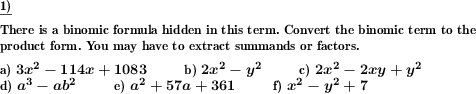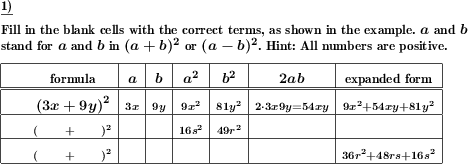Custom math worksheets at your fingertips# Details for problem "Extract binomic formula from term"

Quickname: 1825

Elementary School, Primary School, Junior High School, Middle School, High School.

## Summary

A binomic formula is hidden in a term. Factor out the binomic formular or extract the summand.

## Example## Description

The expanded term for a binomic formula is part of a given term. The given term has been created by multiplying the binomic formular with a factor or by adding a summand or difference. The task is to extract the binomic formula and convert it to the original form written as a factor of two summands. To perform this, either the factor applied has to be factored out or the additional summand has to be extracted.

The summands of the formula's factors will be either a variable and a literal number or both of them will be variables. In no case, both summands will be numbers, which means the expanded term will always contain variables and it does not simplify to a single literal number.

The number of problems is selectable. For the types of formulas that will appear it can be specified that only some of the three binomic formulas will be used. The modifying term can be either a product, a sum or a difference. There is an option to choose with of these arithmetic operations can appear.

Variables that appear will always be named a and b or x and y.

## Free worksheets and solution sheets with answers for download

Download free printable worksheets for this math problem here. The worksheet contains the problems only, the solution sheet includes the answers. Just click on the respective link.

•Worksheet 1Solution sheet with answers
•Worksheet 2Solution sheet with answers
•Worksheet 3Solution sheet with answers

If you can not see the solution sheets for download, they may be filtered out by an ad blocker that you may have installed. If this is the case, please allow ads for this page and reload the page. The solution sheets will then reappear.

• Do these sample worksheets do not really fit?
• Do you need more math worksheets, with a different level of difficulty?
• Would you like to combine different problems on a worksheet and adjust them to your needs?
• As a teacher, you can put together your own worksheets using the automatically generated math problems provided.
With a free initial credit, you can start creating your own math worksheets in a few minutes.

You can try it for free! Register here, to create custom worksheets now!

## Customization options for this problem

Parameter
Possible values
Number of problems
1, 2, 3, 4, 5, 6, 7, 8, 9, 10
Binomic formula type
(a+b)^2, (a-b)^2, (a+b)(a-b), (a+b)^2 & (a-b)^2, all
Part of
product,sum,difference, product, sum, sum,difference
Other term
variable,number, variable, number
Provide hint
Yes, No

## Similar problems

Remark
Description
Name and direct link
Factorisation of a generic term
Factorise a term with variables
Opposite problem
Simplify a term which contains a binomic formula

## dw-Math worksheet templates that do contain this problem

Name
Title
Description
Binomic formulas: Advanced applications
Use the binomic formulas to simplify and expand terms.

## Other types of problems that appear on worksheets with this problem:

Relevance
Name
Description
Quickname
Example
****
Expand and simplify binomic formula, advanced
Apply binomic formula expansion rules to simplify term. Advanced version mit multiples of variables as summands.****
Simplify term with binomic formula, combine like terms
Simplify a term which contains a binomic formula****
Examine the elements of binomic formulas
Elements of a binomic formula and their expanded form are grouped in a table. Fill the empty spaces.You may pass on the worksheets offered for download on this page to others, but not modify or sell them.